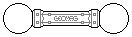Web textodigital.com
Concentric cuboctahedra
It is very easy to build a rhombitruncated cuboctahedron around a rhombicuboctahedron.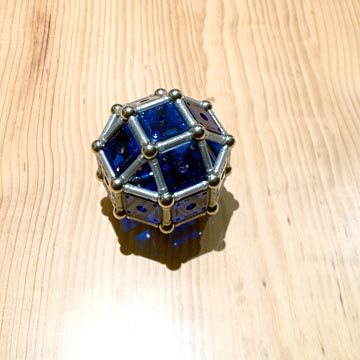Construction of the rhombitruncated cuboctahedron around the rhombicuboctahedron, step 1: rhombicuboctahedron
One can choose between building twelve cubes on the square faces which share edges with triangular faces (option A, left-hand picture), or building eight cupolas on the triangular faces (option B, right-hand picture):Construction of the rhombitruncated cuboctahedron around the rhombicuboctahedron, step 2, option A: cubes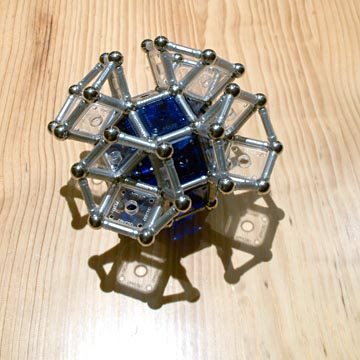Construction of the rhombitruncated cuboctahedron around the rhombicuboctahedron, step 2, option B: triangular cupolas
In both cases, the only remaining edges are four alternate ones for each of the six octagonal faces: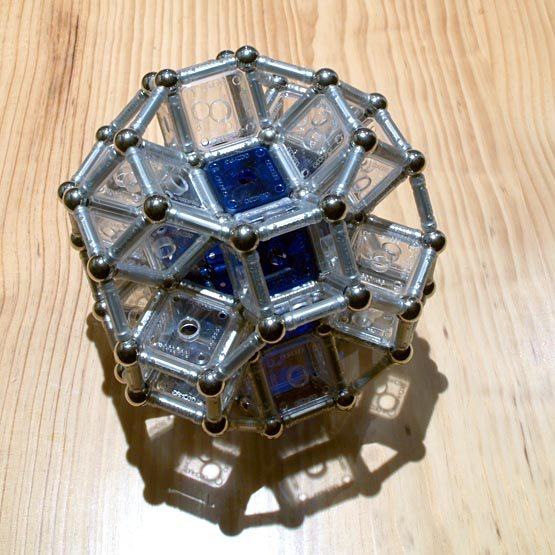Rhombitruncated cuboctahedron around the rhombicuboctahedron 306 pieces: 72 balls, 168 rods, 66 squares (1.53 kg)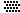The hollow hexagonal faces could be reinforced by means of six rods or three rods and three rhombuses. For bigger strength, a regular tetrahedron could be built on each of the triangular faces of the original rhombicuboctahedron; its upper vertex is the same as the center ball in the hexagon:Rhombitruncated cuboctahedron around the rhombicuboctahedron: reinforced hexagon 1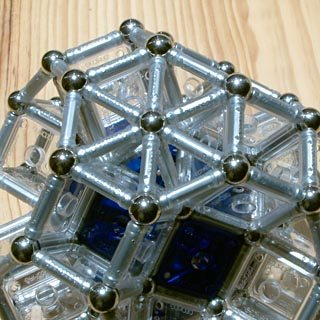Rhombitruncated cuboctahedron around the rhombicuboctahedron: reinforced hexagon 2
Lastly, twelve square panels could be added in order to close the cubes built on the faces of the inner rhombicuboctahedron:Reinforced rhombitruncated cuboctahedron 398 pieces: 80 balls, 240 rods, 78 squares (1.96 kg)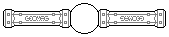Because of its geometry, the rhombitruncated cuboctahedron can be used to build giant models of the tetrahedron, the cube, and the octahedron. In these cases, we will use the reinforced version.